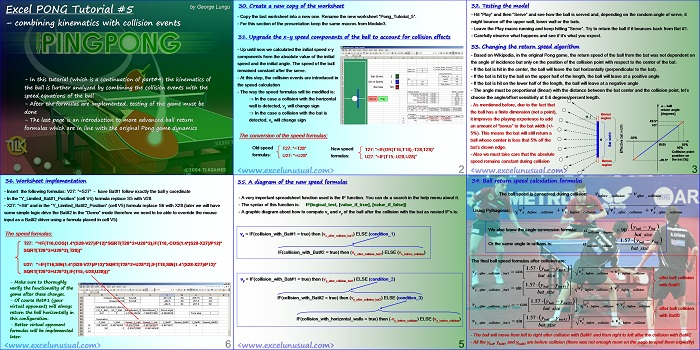# How to make a game of PONG in Excel – part #5

In this part of the tutorial, the analysis of the ball movement is continued. The effects of the collision events are introduced in the equations of movement.[sociallocker][/sociallocker]

## Excel PONG Tutorial #5

by George Lungu

– combining kinematics with collision events
– In this tutorial (which is a continuation of part#4) the kinematics of
the ball is further analyzed by combining the collision events with the
speed equations of the ball
– After the formulas are implemented, testing of the game must be
done
– The last page is an introduction to more advanced ball return
formulas which are in line with the original Pong game dynamics

### 30. Create a new copy of the worksheet

– Copy the last worksheet into a new one. Rename the new worksheet “Pong_Tutorial_5”.

– For this section of the presentation keep the same macros from Module3.

### 31. Upgrade the x-y speed components of the ball to account for collision effects

– Up until now we calculated the initial speed x-y
components from the absolute value of the initial
speed and the initial angle. The speed of the ball
remained constant after the serve.

– At this step, the collision events are introduced in
the speed calculation

– The way the speed formulas will be modified is:
-In the case a collision with the horizontal
wall is detected, vy will change sign
– In the case a collision with the bat is
detected, vx will change sign

### The conversion of the speed formulas:

Old speed formula:  T27: “=T28”, U27: “=U28”

New speed formulas T27: “=IF(OR(T16,T18),-T28,T28)”, U27: “=IF(T15,-U28,U28)”

<www.excelunusual.com> 2

### 32. Testing the model

– Hit “Play” and then “Serve” and see how the ball is served and, depending on the random angle of serve, it
might bounce off the upper wall, lower wall or the bats.

– Leave the Play macro running and keep hitting “Serve”. Try to return the ball if it bounces back from Bat #1.

– Carefully observe what happens and see if it’s what you expect.

### 33. Changing the return speed algorithm

– Based on Wikipedia, in the original Pong game, the return speed of the ball from the bat was not dependent on
the angle of incidence but only on the position of the collision point with respect to the center of the bat.

– If the bat is hit in the center, the ball will leave the bat horizontally (perpendicular to the bat).

– If the bat is hit by the ball on the upper half of the length, the ball will leave at a positive angle

– If the bat is hit on the lower half of the length, the ball will leave at a negative angle

– The angle must be proportional (linear) with the distance between the bat center and the collision point, let’s
choose the angle/offset sensitivity at 0.6 degrees/percent length.

-As mentioned before, due to the fact that the ball has a finite dimension (not a point), it improves the playing experience to add an amount of “bonus” to the bat width (+/-5%).

-This means the bat will still return a ball whose centeris less that 5% off the bat’s drawn edge.

-Also we must take care that the absolute speed remains constant during collision

<www.excelunusual.com> 3

### 34. Ball return speed calculation formulas

The ball speed is conserved during collision: v *v
before collision after collision

Using Pythagoras:

Or the same angle in radians is:  1.57

The final ball speed formulas after collision are:

– The ball will move from left to right after collision with Bat#1 and from right to left after the collision with Bat#2
– All the yball, ybat#1 and ybat#2 are before collision (there was not enough room on the page to spell them
explicitly)

### 35. A diagram of the new speed formulas

– A very important spreadsheet function used is the IF function. You can do a search in the help menu about it.

– The syntax of this function is: IF(logical_test, [value_if_true], [value_if_false])

– A graphic diagram about how to compute vx and vy of the ball after the collision with the bat as nested IF’s is:

v_x = IF(collision_with_Bat#1 = true) then (vx_after_colision_bat1) ELSE (condition_1)

IF(collision_with_Bat#2 = true) then (vx_after_colision_bat2) ELSE (vx_before_colision)

v_y = IF(collision_with_Bat#1 = true) then (vy_after_colision_bat1) ELSE (condition_2)

IF(collision_with_Bat#2 = true) then (vy_after_colision_bat2) ELSE (condition_3)

IF(collision_with_horizontal_walls = true) then (-vy_before_colision) ELSE (vy_before_colision)

<www.excelunusual.com> 5

### 36. Worksheet implementation

– Insert the following formulas: V27: “=S27” – have Bat#1 follow exactly the ball y coordinate

– In the “Y_Limited_Bat#1_Position” (cell V4) formula replace S5 with V28

– X27: “=S6” and in the “Y_Limited_Bat#2_Position” (cell V5) formula replace S6 with X28 (later we will have
some simple logic drive the Bat#2 in the “Demo” mode therefore we need to be able to override the mouse
input as a Bat#2 driver using a formula placed in cell V5)

The speed formulas:
T27: “=IF(T16,COS(1.4*(S28-V27)/P12)*SQRT(T28^2+U28^2),IF(T18,-COS(1.4*(S28-X27)/P12)*
SQRT(T28^2+U28^2),T28))”
U27: “=IF(T16,SIN(1.4*(S28-V27)/P12)*SQRT(T28^2+U28^2),IF(T18,SIN(1.4*(S28-X27)/P12)*
SQRT(T28^2+U28^2),IF(T15,-U28,U28)))”

– Make sure to thoroughly
verify the functionality of the
game after these changes.

– Of course Bat#1 (your
virtual opponent) will always
return the ball horizontally in
this configuration.

– Better virtual opponent
formulas will be implemented
later.

by George Lungu <www.excelunusual.com>